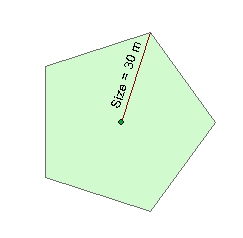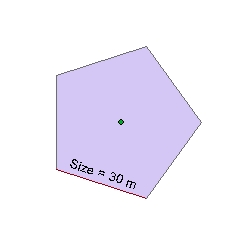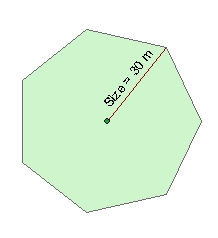Go to ET GeoWizards 12.x User Guide

Points To Regular Polygons

Go to ToolBox Implementation

Creates regular polygons from points in a point dataset and user defined number of sides, size and rotation angle. The source point will be located in the center of the polygons.

Inputs:

• A Point feature class
• Number of sides of the polygons to be created.
• Size represents option - depending on the user input the size parameter can represent
• The side of the polygon
• The radius of the circle inscribed in the polygon
• The radius of the circle circumscribed around the polygon.
• Size of the polygon. The size can be fixed for all points or different assigned from the values in a numeric field of the point attribute table
• Rotation angle.

Outputs:

• New polygon feature class. All the original field values will be transferred from the points to the polygons.

Notes:

• The values for the size should be in the units of the spatial reference of the input Point dataset
• The angle (if used) should be in Decimal Degrees and have Polar orientation - East = 0, anti-clockwise. The angle defines the location of the start vertex of the polygon.Examples:

 Size = 30 , Angle = 0, Number sides = 5 Option - Radius Inscribed Size = 30 , Angle = 0, Number sides = 5 Option - Radius Circumscribed Size = 30 , Angle = 0, Number sides = 5 Option - SideSize = 30 , Angle = 0, Number sides = 6Option - Radius Circumscribed Size = 30 , Angle = 0, Number sides = 7Option - Radius Circumscribed Size = 30 , Angle = 0, Number sides = 8 Option - Radius CircumscribedCommand line syntax

ET_GPPointsToRegularPolygons <input_dataset> <out_feature_class> <number_sides>  <Radius In | Radius Out | Side> <Size> <size_field> <rotation_angle>

Parameters

Expression Explanation
<input_dataset> A Point feature class or feature layer
<out_feature_class> A String - the full name of the output feature class (A feature class with the same full name should not exist)
<number_sides> An Integer defining the number of sides of the polygons to be created.
<Radius In | Radius Out | Side> Required. A String indicating the meaning of the <SIZE> parameter